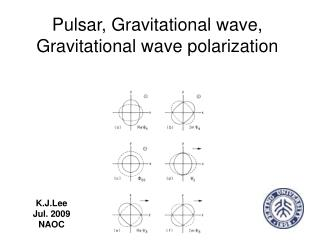DownloadDownload PresentationPulsar, Gravitational wave, Gravitational wave polarization

# Pulsar, Gravitational wave, Gravitational wave polarization

Télécharger la présentation## Pulsar, Gravitational wave, Gravitational wave polarization

- - - - - - - - - - - - - - - - - - - - - - - - - - - E N D - - - - - - - - - - - - - - - - - - - - - - - - - - -
##### Presentation Transcript

1. Pulsar, Gravitational wave, Gravitational wave polarization K.J.Lee Jul. 2009 NAOC

2. Outline • Gravity theory, Spacetime geometry • Gravitational Wave and its polarization • Pulsar, Pulsar timing signal • Detect G-wave using pulsar • Present uplimit • Can we say GR is correct?

3. Gravity theory, Spacetime geometry

4. Gravity theory, geometry of spacetime • Gravity theory must be a geometrical theory, even from Newton times.

5. Gravitational wave Gravitation = Geometry Gravitational wave = Geometrical wave

6. A little bit math In the vacuum • You get the wave. But things are more complex than this. • One important issues is the gauge

7. G-wave, a toy of Einstein • The idea appears very soon after the GR is found • Another very serious mistake of Einstein

8. The TT gauge for GR The meaning of TT gauge is when wave transmit along Z direction we solution looks like How G-wave plays ball (test particles)?

9. Non-Einsteinian theories? Ap At Ae Ab Al 1972, Eardley et al.

10. G-wave is G-wave y x y x

11. Some ground based and space base efforts.

12. Pulsar, Pulsar timing signal

13. What is pulsar • Here, you can just regard pulsar as an exremely accurate clock radiating photons (radio wave) according to the accurate timing. • The photons are broad band Thompson et al.

14. Pulsar spin is slowing down 2 1 3 4

15. In reality... In fact TOA is more complex than I have illustrated....

16. We have so many accurate clock • We can set up time standard • We can check NIST atomic clock • We can check solar system dynamics • We can do deep space guiding system • We can....

17. Gravitational wave detection using pulsar timing

18. The optical theory in curved space time Geometric optic

19. Timing formula

20. The First Possibility As So we can test whether there is some gravitational wave structure. We can not discreminate noise vs signal People have already done something like that (similar to doppler sitellite tracking....)

21. Single pulsar response function Longitudinal?!! To understand this back to geodesic equation

22. Something like Landau-damping

23. Uplimits for non-Einsteinian modes We find the link formula

24. Uplimts for a lazy man Just use the link formula to convert GR uplimit (Jenet et al. 2006) to non-Einsteinian modes. Lee et al. 2009 b

25. 2nd Possibility Correlation function Correlate the two R(t) Define C=<R(t,P1) R(t,P2)> Why?

26. Pulsar correlation and gravitational wave HD function

27. Gravitational wave detection • We can check the existence for angular dependent correlation to make sure we detect gravitational wave. • The details statistics are complex. • Non-Einsteinian modes?

28. GR B Longitudinal Shear Mode dependent polarization Lee et al. 2009a

29. Celestial object Detection procedure Receiver TEMPO . . . . . Compare

30. The detection significance

31. The Probability Intrusion No intrusion Alarm √ X False alarm rate (pf) No Alarm X Missing rate (Pm)‏ √ The ability to discriminate them Neyman-Person type detection Example: Radar detect enemy’s intrusion Given a false alarm (1e-3) rate we calculate the missing rate to check the quality of discriminating processes. It clearly that if the missing rate is 50%, we have average ability to give 3-sigma detection, if noise is Gaussian.

32. The ability to discriminate them 1 20 yr 10 yr 5 yr 20 yr 10 yr 5 yr 30ns 100ns L S B L S B

33. The ability to discriminate them 2 20 yr 10 yr 5 yr 20 yr 10 yr 5 yr 30ns 100ns L S B L S B

34. The ability to discriminate them 3 20 yr 10 yr 5 yr 20 yr 10 yr 5 yr 30ns 100ns L S B

35. Why we do this? • General relativity is just one of theories. • We have more than one hundred theories of gravity. • Which one is correct?

36. What we will achieve? • We develop the mathematical presentation for the polarization of GW for general metric theory • We figure out how the pulsar timing response to GW with different polarization modes • We build up the detection algorithm • We evaluate the detector’s quality • With 40 pulsars we can detect GW stronger than hc~1e-14, 1e-14 for GR and B modes; With 60 pulsars, shear and longitudinal modes stronger than hc~1e-16 and 3e-17 can be detected. • With 40, 100, and 300 pulsars, we can discriminate the modes with 90% probability given false alarm rate 0.001. • Test gravity theory via gravitational wave observation directly.

37. Why we need wave-type test? • Classical Test (h<<1, v/c<<1) • Weak field and low speed • Relativistic Binary Pulsar Test (v/c<<!) • strong field and low speed • Wave field test (h<<1) • Weak field and high speed

38. Why Wave field test? • One wrong idea: Post Newtonian test is everything. • But if you want to use PN test to investigate gravitational wave or graviton spin, you will need expansion to infinite order, because the PN is expansion respected to (v/c). • Thus G-wave test is independent from PN.

39. Conclusion • Gravitational wave polarization are physically interesting • Pulsar timing is a good tool to measure G-wave polarization. • Pulsar timing is better than LISA for longitudinal modes. • Gravitational wave pulsar timing signal processing techniques are important for many application (time scale, navigation, solar system dynamics et al.).

40. Thanks! BTW, wake up the guy beside you.